This is my reading note for Multi-head or Single-head? An Empirical Comparison for Transformer Training. This paper shows that multi head attention is the same as deeper single head attention, but the later is more direct to train and need special care to initialize.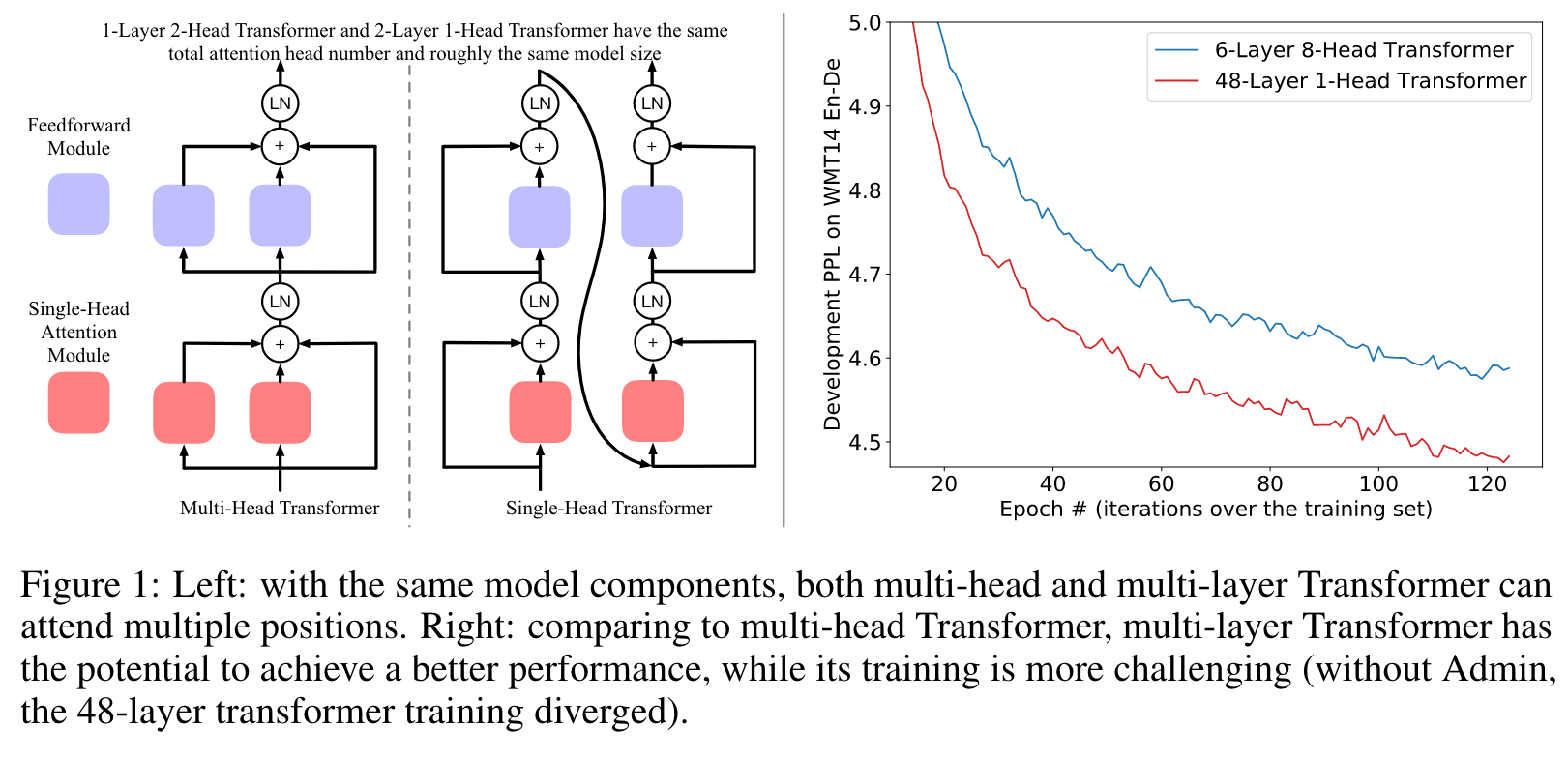# Introduction

The popular belief is that this effectiveness stems from the ability of jointly attending multiple positions. In this paper, we first demonstrate that jointly attending multiple positions is not a unique feature of multi-head attention, as multi-layer single-head attention also attends multiple positions and is more effective. Then, we suggest the main advantage of the multi-head attention is the training stability, since it has less number of layers than the single-head attention, when attending the same number of positions (p. 1)

For example, on machine translation benchmarks, Recurrent Neural Networks (RNNs) can outperform Transformers when both are using the multi-head encoder-decoder attention, and would underperform without using the multi-head attention [Chen et al., 2018]. (p. 1)

as in Figure 1, a multi-head attention module can be viewed as an ensemble model, which combines multiple single-head attention modules by calculating their average. Thus, by integrating these modules differently, we can reconstruct a Transformer to be single-head1 and substantially deeper. These two networks can attend the same number of places (i.e., have the same total number of attention heads ), have roughly the same number of parameters and inference computation complexity, while the multi-head one is shallower and the single-head one is deeper. (p. 2)

# Related Work

To calculate the output for a token in the target sequence, the attention module would calculate a weighted average of source token representations, while the weight is calculated by applying softmax on attention scores. (p. 2)

While these modules can only attend one position in one layer, multi-head attention is developed to improve the conventional attention module by allowing the module jointly attending multiple positions [Vaswani et al., 2017], which is identified as one major reason behind the success of Transformer [Chen et al., 2018]. (p. 3)

# Transformer Architecture

Layer Norm. Layer norm [Ba et al., 2016] plays a vital role in the Transformer architecture. It is defined as fLN(x) = γ x−µ σ + ν, where µ and σ are the mean and standard deviation of x, γ and ν are learnable parameters. (p. 3)

Feedforward. Transformers use two-layer perceptrons as feedforward networks, i.e., fFFN(x) = φ(xW(1))W(2), where W(·) are parameters, and φ(·) is the non-linear function. Specifically, the original Transformer ReLU as the activation function, while later study uses other types of activation function, e.g., BERT uses GELU as the activation function [Hendrycks and Gimpel, 2016]. (p. 3)

Attention. Transformers use the multi-head attention to capture the dependency among input tokens, which is based on the scaled dot-product attention. Scaled dot-product attention tries to query information from the source sequence that is relevant to the target sequence. (p. 3)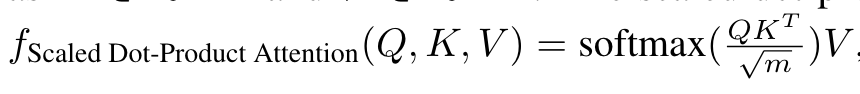One scaled dot-product attention is believed to attend only one position in each row (for each target token), since the output of softmax typically would have one dimension significantly larger than other dimensions in each row. Multi-head attention was proposed to jointly attend multiple positions, which employs multiple scaled dot-product attention in parallel. (p. 3)

Here, we first show that the multi-head attention sub-layers and the feedforward sub-layers have inherent ensemble structures combining multiple smaller modules (e.g., outputs of 8-head attention is the sum of 8 single-head attention). (p. 4)

## Inherent Ensemble Structure within Transformer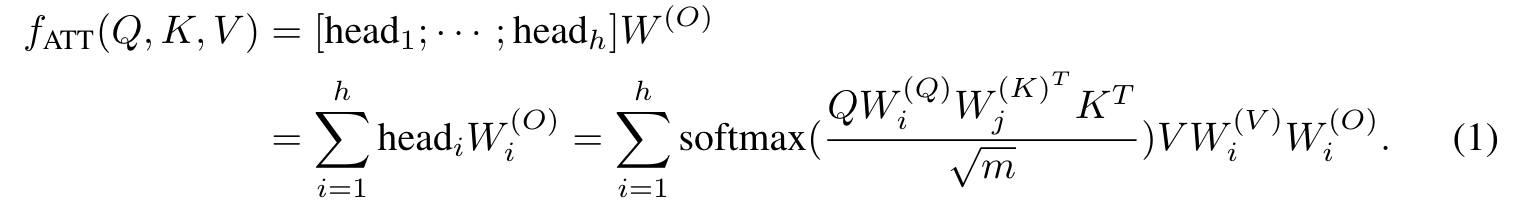Note that on the right side of Equation 1, each term can be viewed as a low-rank version of the general attention [Luong et al., 2015]. Thus, the multi-head attention can be viewed as jointly attending multiple places by ensembling multiple conventional attention modules. (p. 4)

Average Ensemble. Each Transformer sub-layer calculates outputs as fLN(x + f(x)), where f(·) could be fFFN(·) and fATT(·). Thus, the sum calculated in Equation 1 and 3 would be normalized by Var[x + f(x)]. In this way, the joint effect of layer norm and the sum would be similar to combining these modules in an average ensemble manner. (p. 5)

In the original multi-head Transformer, modules in the same layer are combined in an ensemble manner and cannot enhance each other. For example, as in Figure 1, when constructed in the multi- head manner, the two attention heads would have the same input and are agnostic to each other. In this way, the second attention head cannot leverage or benefit from the information captured by the first attention head.

Intuitively, it could be beneficial to allow the second attention head standing on the shoulders of the first attention head. To this end, we integrate these modules differently, and reconstruct the shallow multi-head Transformer into the deep single-head Transformer (As in Figure 1). Note that both models have the same total number of attention heads, roughly same model size, and roughly the same inference computation complexity. (p. 5)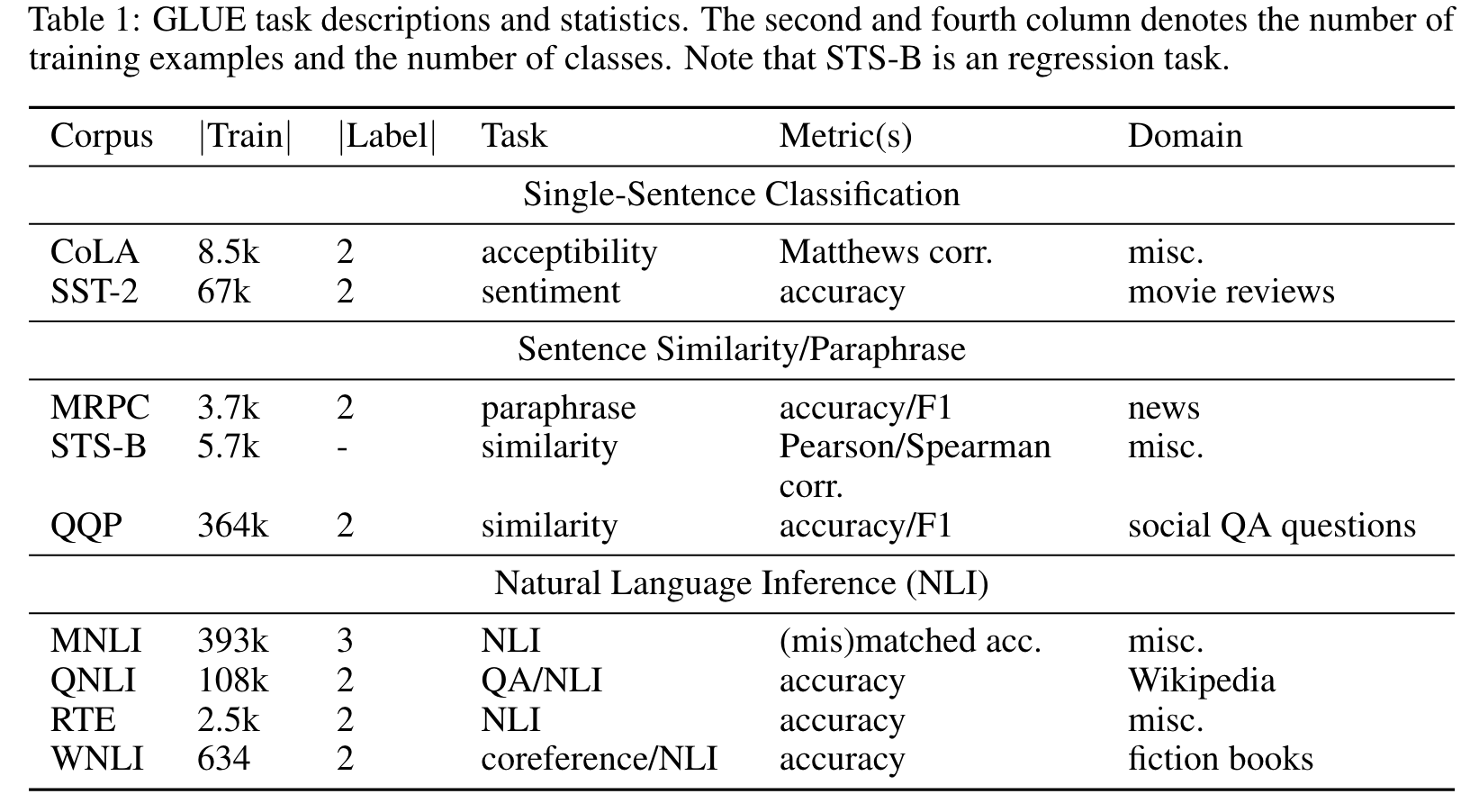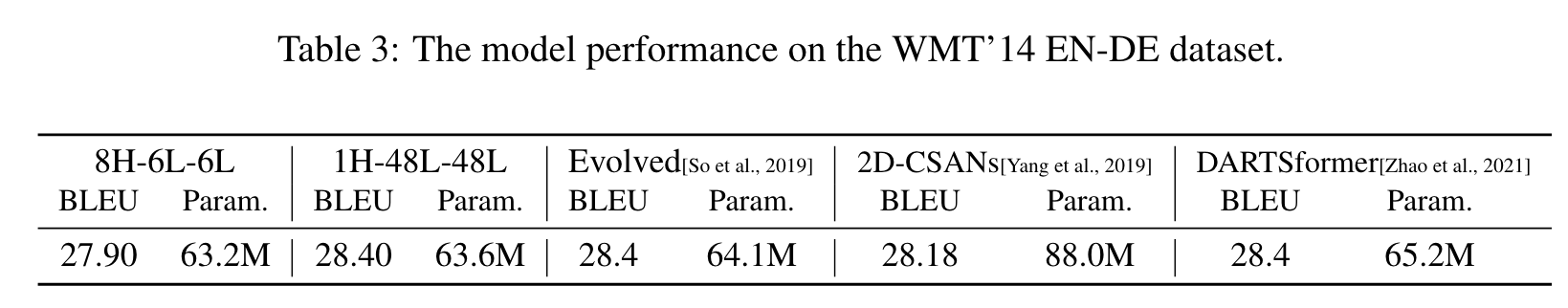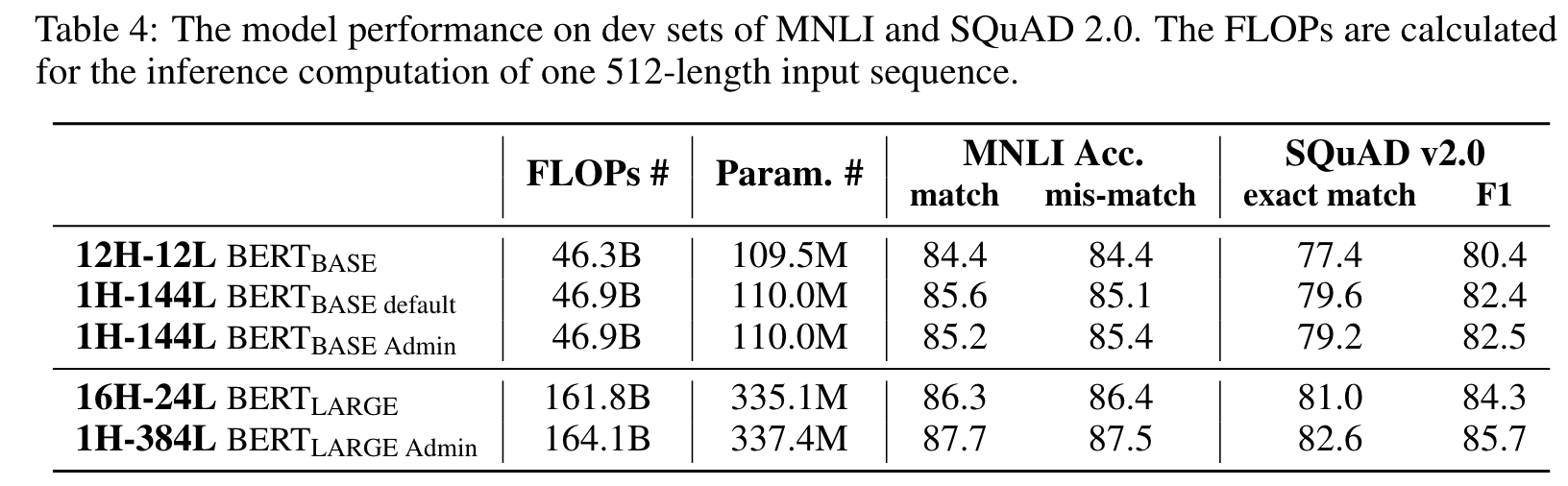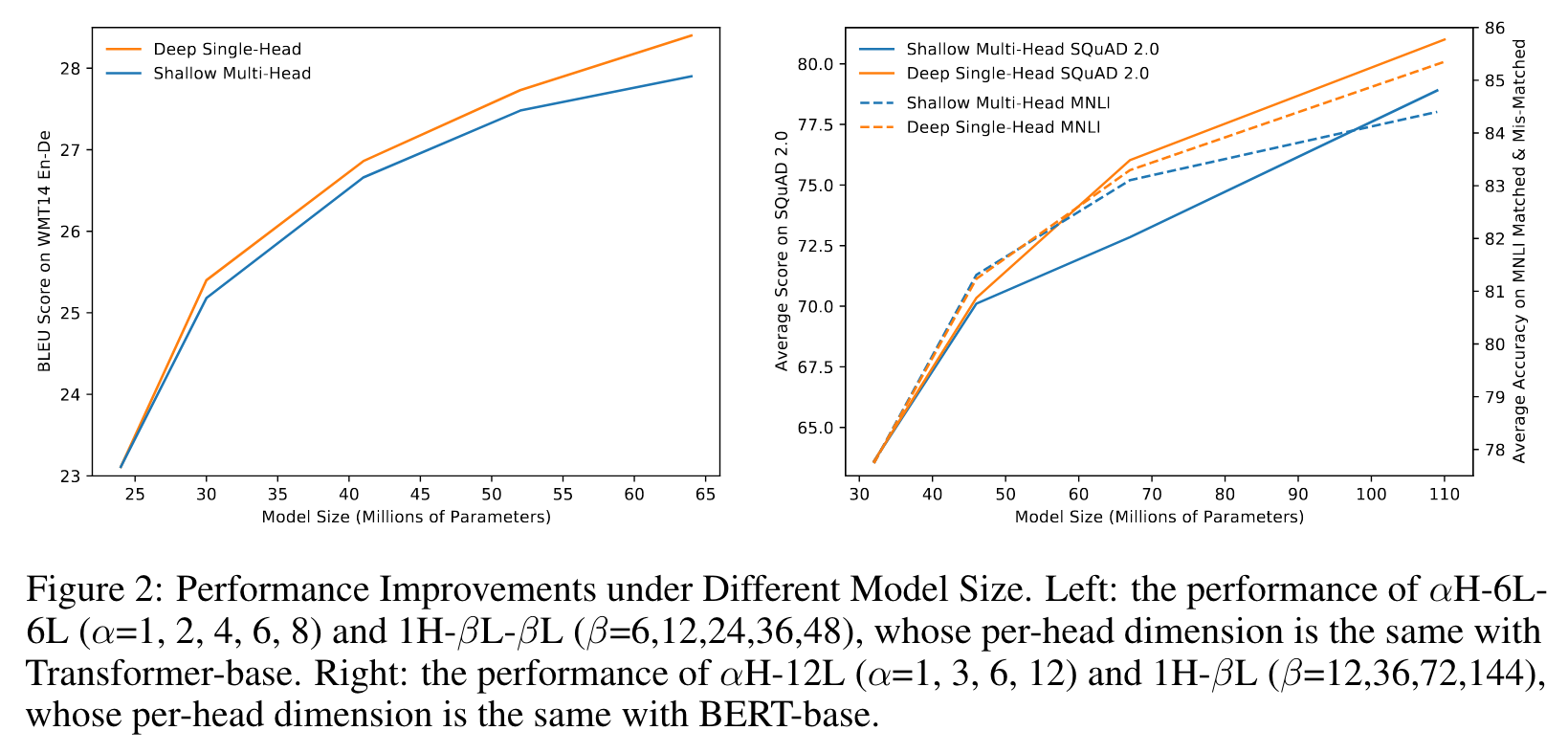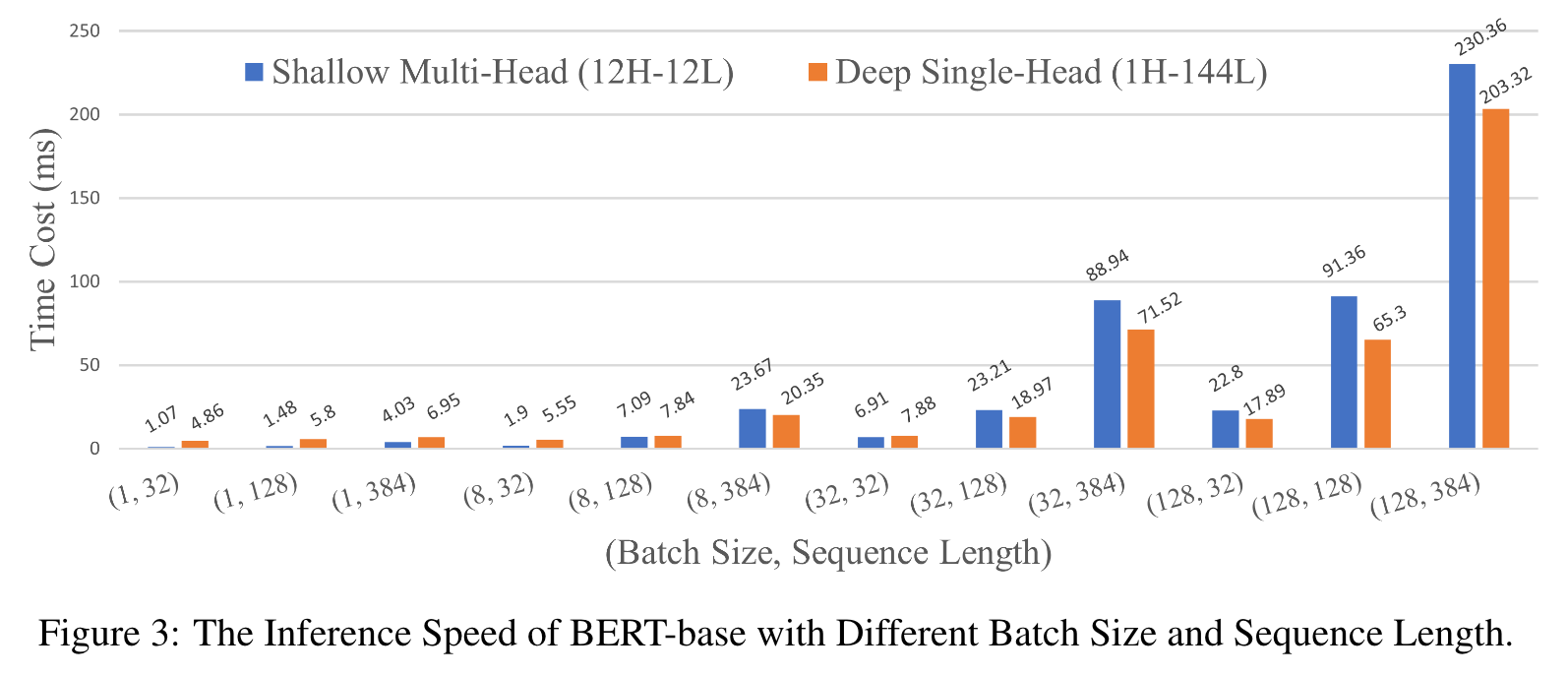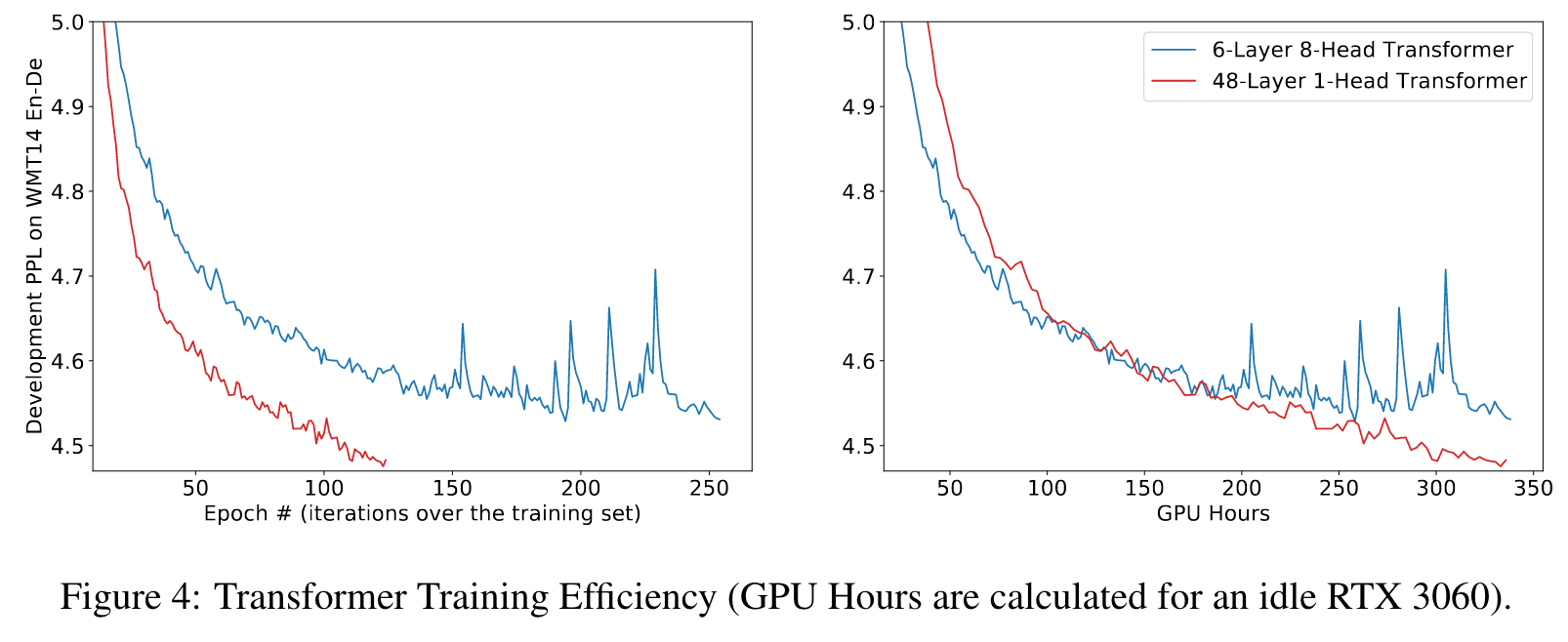## Stability Comparison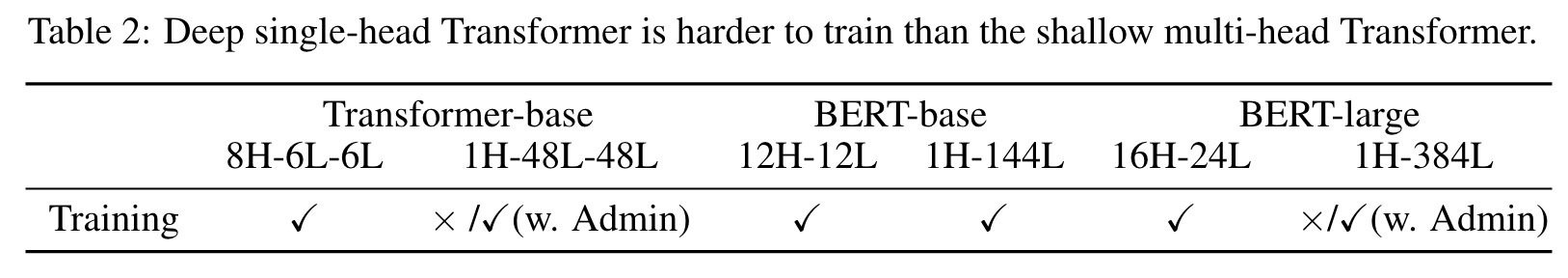As in Table 2, after changing the shallow multi-head Transformer to the deep single-head Transformer, the training fails to converge well for 2 out of 3 models. Note that, although the 1H-144L BERT-base model converges successfully, the model is sensitive to the choice of initialization. (p. 7)

Meanwhile, we show that, with the recent advances in deep learning, the training can be successfully stabilized by Adaptive Model Initialization (Admin), without changing any hyper-parameters Liu et al. [2020b]. (p. 7)

Written on June 30, 2023